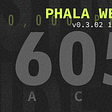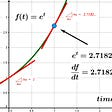# What does 631764 and 975330866421 have in common?

## Introduction to the Kaprekar numbers

As most of you guessed correctly, these two numbers have more in common than just being numbers or that they both include the digit 6 two times.

These numbers are a part of a set called ‘Fixed points of the Kaprekar mapping’.

In order for you to understand what does it actually mean, let’s go through a simple example.
Meet the number 495, he is also a part of the same set, we will work with him to understand why is he exactly a ‘fixed point’.

From the number 495, we will construct two different numbers.
The first number will be constructed from arranging the digits of 495 in descending order and the second number from arranging the digits of 495 in ascending order.
If you followed, the numbers that you should get are 954 and 459.
Note that if we substract these numbers we get our original number, 954–459 = 495.
And that’s why 495 is considered a ‘fixed point’ in relation to the method I described (meaning that if we apply this method on this number it goes back to himself).

While explaining the terminology of a ‘fixed point’ I actually also explained what is the Kaprekar mapping.
The Kaprekar mapping is the method of taking a number and constructing two numbers from it (as we did before) and finally substracting them.

After we understood that, let’s see why it is so unique.
I’ll pick a random 3 digit number, 651 is random enough I guess.
If we apply the Kaprekar mapping to 651 we get the numbers 156 and 651,
651–156 = 495.
We got to our fixed point after just one iteration of the mapping.

The beautiful thing about this method is that if you pick any number with 3 digits with at least two different digits (exceptions are 111, 222…) you will eventually get to 495 after applying the mapping over and over again, and it’s not exclusive to 3 digits numbers and 495 -there is a whole set of numbers that maintains the same feature.

For example, let’s try this with 4 digits number — I’ll randomly choose 5581.
From 5581 we construct 1558, 8551.
8551–1558 = 6993.
From 6993 we construct 3699, 9963.
9963–3699 = 6264.
From 6264 we construct 2466, 6642.
6642–2466 = 4176.
From 4176 we construct 1467, 7641.
7641–1467 = 6174.
From 6174 we construct 1467, 7641.
7641–1467 = 6174.

As you can see we have reached a fixed point- from now on we will get the same value from the mapping, so 6147 also belong to the set.

I have written a small script for you If you want to try it yourself

`n = input('Enter a number: ')prev_n = 0iter = 0while prev_n != n:    iter += 1    x1 = sorted(n)    x2 = sorted(n, reverse=True)    xx1 = int(''.join(x1))    xx2 = int(''.join(x2))    print(f'From {n} we construct {xx1}, {xx2}.')    print(f'{xx2}-{xx1} = {xx2-xx1}')    prev_n = n    n = str(xx2-xx1)print(f'Got to fixed point {n} in {iter} iterations')`

For those of you who wanna explore more, the entire set can be found here.
There are some more special cases for the Kaprekar mapping, you can find more information here.

If you enjoyed this one, maybe you will enjoy the following too.

--

--

--

## More from Eliran Turgeman

Software Developer, Writing about Computer Science, Education, Programming, and Math. https://eliran-turgeman.github.io/

Love podcasts or audiobooks? Learn on the go with our new app.

## Vendetta Incentive Program Launched | Phala Weekly v0.3.02 most## Digital Citations — A Paperless Parking Citation System (An All-Amazon Serverless Cloud Solution)## Microservice — Build Relationship among Engineers via API Communication## 💪 Motivation to learn programming## Importance of Conference and Meet-up for developers## Crodo — Lavax Labs , Review and comparison## Understand ConfigMaps = Configure a Pod to Use a ConfigMap — CKA Exam Preparation Series## Eliran Turgeman

Software Developer, Writing about Computer Science, Education, Programming, and Math. https://eliran-turgeman.github.io/

## The Integrating Factor## Notes on the Exponential Function: Growth and Decay : A Differential Equation## How to turn non-recursive formulas into recursive formulas## The Double and Triple Angle Formulas Derivation by de Moivre’s Theorem